# High School Math : Rectangles

## Example Questions

### Example Question #2 : How To Find The Length Of The Side Of A Rectangle

A rectangle is x inches long and 3x inches wide.  If the area of the rectangle is 108, what is the value of x?

8

12

6

3

4

6

Explanation:

Solve for x

Area of a rectangle A = lw = x(3x) = 3x2 = 108

x2 = 36

x = 6

### Example Question #3 : How To Find The Length Of The Side Of A Rectangle

If the area of rectangle is 52 meters squared and the perimeter of the same rectangle is 34 meters. What is the length of the larger side of the rectangle if the sides are integers?

14 meters

16 meters

15 meters

12 meters

13 meters

13 meters

Explanation:

Area of a rectangle is = lw

Perimeter = 2(l+w)

We are given 34 = 2(l+w) or 17 = (l+w)

possible combinations of l + w

are 1+16, 2+15, 3+14, 4+13... ect

We are also given the area of the rectangle is 52 meters squared.

Do any of the above combinations when multiplied together= 52 meters squared? yes 4x13 = 52

Therefore the longest side of the rectangle is 13 meters

### Example Question #1 : How To Find The Length Of The Side Of A Rectangle

The width, in cm, of a rectangular fence is 2 more than half its length, in cm. Which of the following gives the width, w cm, in terms of length, l cm, of the rectangular fence?

w = 2l – 2

w = ½ l + 2

w = 2l + 2

w = ½ l – 2

w = ½ l + 2

Explanation:

To find the width, we must take half of the length, which means we must divide the length by 2. Then we must take 2 more than that number, which means we must add 2 to the number. Combining these, we get:

w = ½ l + 2

### Example Question #7 : How To Find The Length Of The Side Of A Rectangle

The width of a rectangle is 2 inches longer than 3 times its length.  Which of the following equations gives the width, w, of the rectangle in terms of its length, l,?

w = 1/3l +2

w = 6l +2

w = 3l + 2

w = 3l – 2

w = 3l + 2

Explanation:

The width equals 3 times the length, so 3l, plus an additional two inches, so + 2, = 3l + 2

### Example Question #1 : How To Find The Length Of The Side Of A Rectangle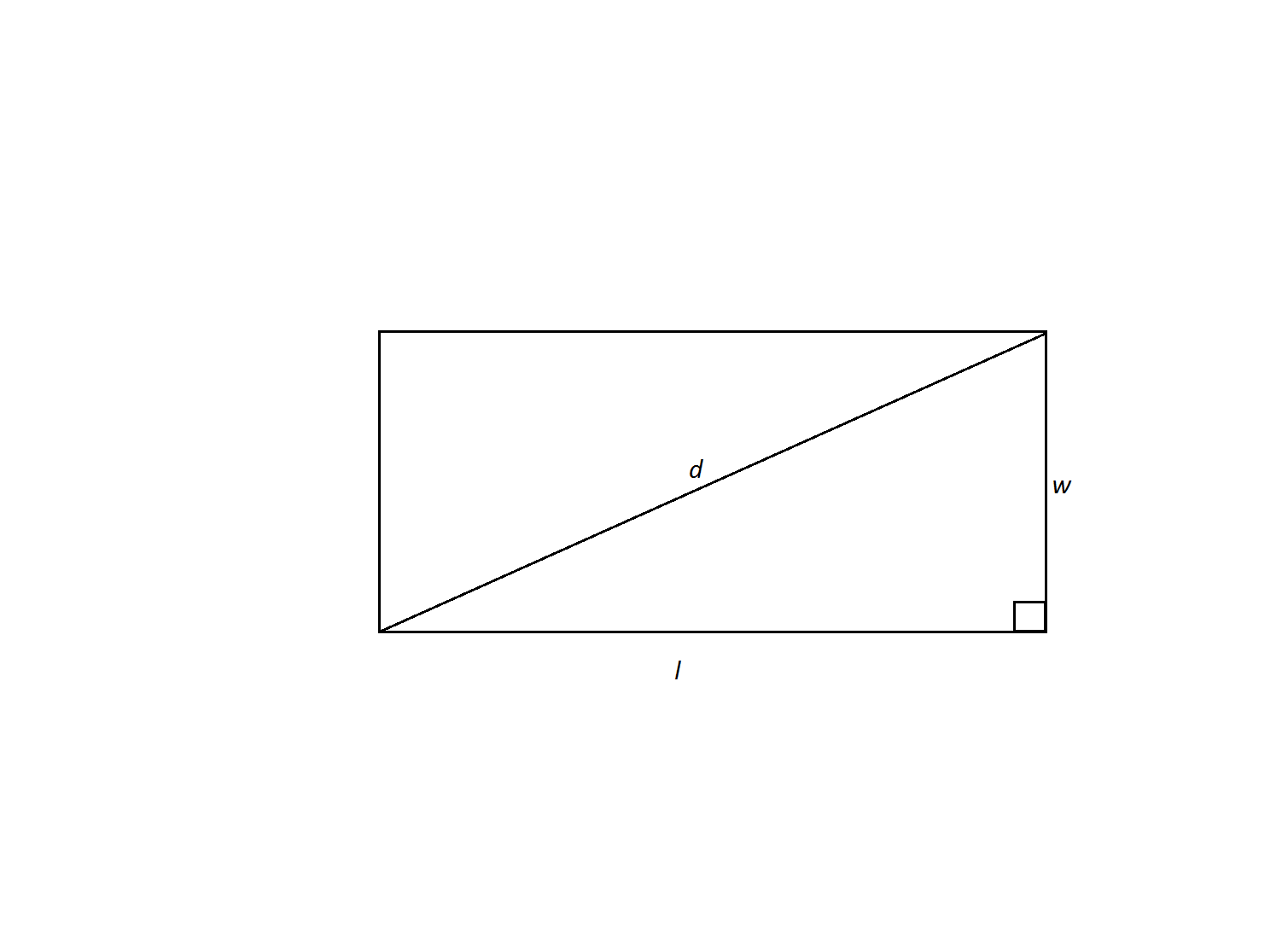A rectangle has a width of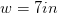and a diagonal of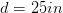. What is its perimeter?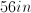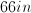Not enough information to solve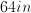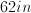Explanation:

We are given the rectangle's diagonal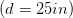and width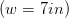and are asked to find its perimeter. The diagonal forms two right triangles within the rectangle; therefore, we can use the Pythagorean theorem to find the length of the rectangle's missing side. Then we can use the formula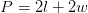to find the perimeter of the rectangle.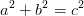In our case, we can rename the variables to match our triangle.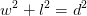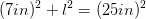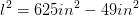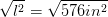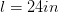Now we can calculate the perimeter.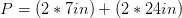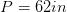### Example Question #8 : How To Find The Length Of The Side Of A Rectangle

Your dad shows you a rectangular scale drawing of your house. The drawing is 6 inches by 8 inches. You're trying to figure out the actual length of the shorter side of the house. If you know the actual length of the longer side is 64 feet, what is the actual length of the shorter side of the house (in feet)?

60

36

48

81

32

48

Explanation:

We can solve this by setting up a proportion and solving for x,the length of the shorter side of the house. If the drawing is scale and is 6 : 8, then the actual house is x : 64. Then we can cross multiply so that 384 = 8x. We then divide by 8 to get x = 48.

### Example Question #1 : How To Find The Length Of The Diagonal Of A Rectangle

What is the diagonal of a rectangle with a side length of 5 and a base of 8? (Round to the nearest tenth)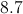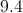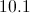Explanation:

To find the diagonal of a rectangle we must use the side lengths to create a 90 degree triangle with side lengths of 5, 8, and a hypotenuse which is equal to the diagonal.

If we have a right angle triangle and a value for two of the three side lengths, we use the Pythagorean Theorem to solve for the length of the third side.

The two side lengths that meet to form the right angle are labeledandwhich are interchangeable for each side length.

The long length connecting them is labeledand is known as the hypotenuse.

The Pythagorean Theorem states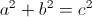Take 5 and 8 and plug them into the equation asandto yield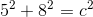First square the numbers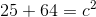After squaring the numbers add them together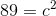Once you have the sum, square root both sides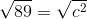After calculating our answer for the diagonal is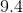.

### Example Question #2 : How To Find The Length Of The Diagonal Of A Rectangle

What is the length of the diagonal of a rectangle that is 3 feet long and 4 feet wide?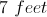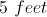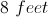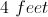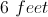Explanation:

The diagonal of the rectangle is equivalent to finding the length of the hypotenuse of a right triangle with sides 3 and 4. Using the Pythagorean Theorem: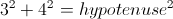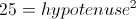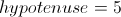Therefore the diagonal of the rectangle is 5 feet.

### Example Question #3 : How To Find The Length Of The Diagonal Of A Rectangle

The length and width of a rectangle are in the ratio of 3:4. If the rectangle has an area of 108 square centimeters, what is the length of the diagonal?

12 centimeters

15 centimeters

9 centimeters

24 centimeters

18 centimeters

15 centimeters

Explanation:

The length and width of the rectangle are in a ratio of 3:4, so the sides can be written as 3x and 4x.

We also know the area, so we write an equation and solve for x:

(3x)(4x) = 12x= 108.

x2 = 9

x = 3

Now we can recalculate the length and the width:

length = 3x = 3(3) = 9 centimeters

width = 4x = 4(3) = 12 centimeters

Using the Pythagorean Theorem we can find the diagonal, c:

length2 + width2 = c2

92 + 12= c2

81 + 144 = c2

225 = c2

= 15 centimeters

### Example Question #6 : Quadrilaterals

A contractor is going to re-tile a rectangular section of the kitchen floor. If the floor is 6ft x 3ft, and he is going to use square tiles with a side of 9in. How many tiles will be needed?

24

40

32

2